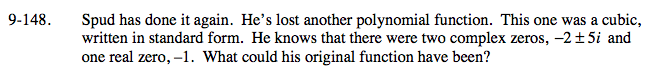### Home > A2C > Chapter Ch9 > Lesson 9.3.2 > Problem9-148

9-148.Use the three roots to find the individual polynomials that make up the cubic equation.

(x + 1)(x + 2 + 5i)(x + 2 − 5i)

Now multiply the two complex polynomials.

(x + 2 + 5i)(x + 2 − 5i) = x2 + 4x + 29

Now multiply this equation by x + 1.

(x + 1)(x2 + 4x + 29)

x3 + 5x2 + 33x + 29